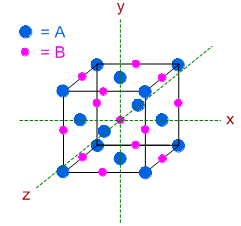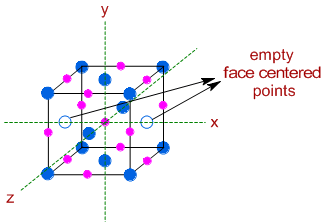JEE  >  Solid State Numerical- Solid State, Physical Chemistry

# Solid State Numerical- Solid State, Physical Chemistry - Notes - JEE

In a solid "AB" having NaCl structure "A" atoms occupy the corners of the cubic unit cell. If all the face-centred atoms along one of the axes are removed, then the resultant stoichiometry of the solid is                            [JEE - 2000]
• a)
AB2
• b)
A2
• c)
A4B3
• d)
A3B4

Ref: https://edurev.in/question/487932/In-a-solid-quotABquot-having-NaCl-structure-quotAquot-atoms-occupy-the-corners-of-the-cubic-unit-cel

 1 Crore+ students have signed up on EduRev. Have you?

'AB' has NaCl structure, otherwise known as Rock salt structure. Hence one type of atoms must be arranged in FCC pattern (at all the 8 corners and all the 6 face centers) and other type of atoms must be present on all the 12 edges as well as at the center of the unit cell.

Since, 'A' atoms are occupying the corners of unit cell, they must also be arranged on the face centers. The B atoms can be found at the 12 edges and at the center of the unit cell as shown below.Therefore, before removing face centered atoms:

The number of 'A' atoms = (8 x 1/8) + (6 x 1/2) = 1 + 3 = 4 [there are 8 'A' atoms at the corners and 6 'A' atoms at the face centers]

The number of 'B' atoms = (12 x 1/4) + (1 x 1) = 3 + 1 = 4 [there are 12 'B' atoms at the edges and 1 'B' atom at the center of the unit cell]

However after removing two face centered 'A' atoms along one of the axes (x axis) as shown below :The number of 'A' atoms = (8 x 1/8) + (4 x 1/2) = 1 + 2 = 3 [there are now only 4 'A' atoms at the face centers]

The number of 'B' atoms = (12 x 1/4) + (1 x 1) = 3 + 1 = 4

Therefore the stoichiometry after removing these atoms is: A3B4

Note:

Each atom at the corner contributes 1/8th, and each atom at the face center contributes 1/2nd and each atom along the edge contributes 1/4th to the unit cell.

The document Solid State Numerical- Solid State, Physical Chemistry - Notes - JEE is a part of JEE category.
All you need of JEE at this link: JEE
 Use Code STAYHOME200 and get INR 200 additional OFF

Track your progress, build streaks, highlight & save important lessons and more!

,

,

,

,

,

,

,

,

,

,

,

,

,

,

,

,

,

,

,

,

,

,

,

,

;